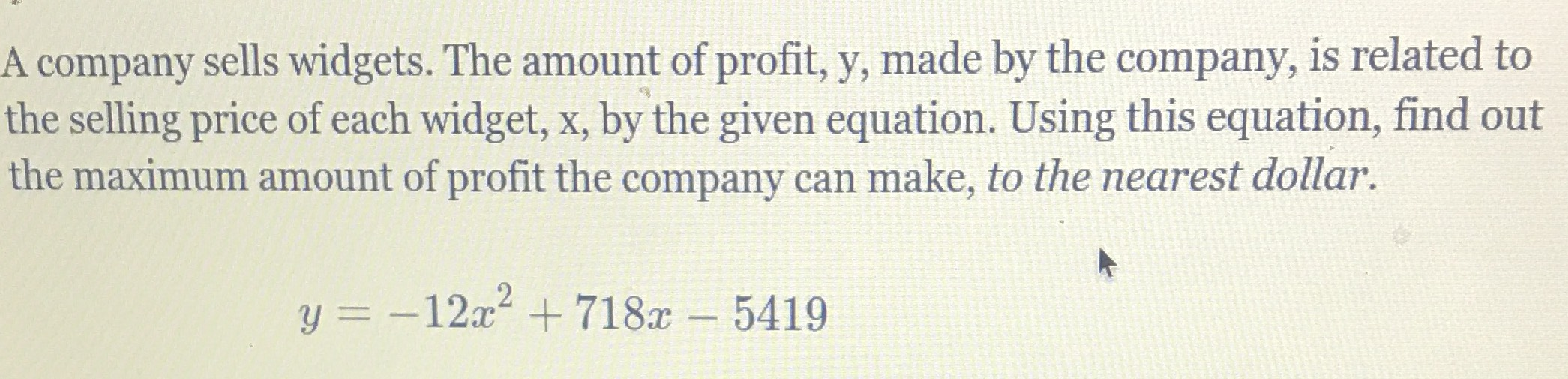### ¿Todavía tienes preguntas de matemáticas?

Pregunte a nuestros tutores expertos
Algebra
PreguntaA company sells widgets. The amount of profit, y, made by the company, is related to the selling price of each widget, $$x$$ , by the given equation. Using this equation, find out the maximum amount of profit the company can make, to the nearest dollar.

$$y = - 12 x ^ { 2 } + 718 x - 5419$$